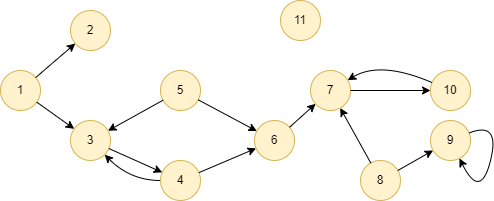# Change Nickname

Current Nickname:

Certifications

Certificate Issued at Valid until Serial No. File
Serial No. Valid until

Not having one? Apply now! >>>

Invoice

ProductName CreateTime ID Price File
ProductName CreateTime ID Price File

# Node2Vec Walk

## Overview

Unlike the classic random walk, Node2Vec random walk adopts a second-order random walk and controls whether the walk is biased toward BFS or DFS through parameters. The sequences generated by the Node2Vec Walk algorithm are used as samples for the Node2Vec graph embedding algorithm, as detailed in the chapter Node2Vec.

## Results and Statistics

Perform Node2Vec random walk in the graph below for 3 times, start from node 3 with a depth of 5, each edge weight is 1, set p as 10000 and q as 0.01:Algorithm results: 3 node arrays are contained in the returned `walks`

walks
[3, 5, 3, 1, 2]
[3, 4, 6, 7, 8]
[3, 1, 2, 1, 3]

Algorithm statistics: N/A

## Command and Configuration

• Command：`algo(random_walk_node2vec)`
• Configurations for the parameter `params()`:
Name Type
Default
Specification
Description
ids / uuids []`_id` / []`_uuid` / / IDs or UUIDs of nodes to start the walk; all nodes to be selected if not set
walk_length int 1 >=1 Depth of each walk, i.e. the number of nodes walking through
walk_num int 1 >=1 Number of walks
p float 1 >0 `return` parameter; the larger the value, the smaller the probability of returning
q float 1 >0 `in-out` parameter that represents the probability of being to walk far away; >1 means tend to walk at the same level, >1 means tend to walk far away
edge_schema_property []`@<schema>?.<property>` / Numeric edge property, LTE needed Edge weight property/properties, schema can be either carried or not; nodes only walk along edges with the specified properties and the probability of passing through these edges is proportional to the edge weight; if edge has multiple specified properties, the edge weight is the sum of these property values; the weight of all edges is 1 if not set
buffer_size int 1000 / Number of results to return; < 0 means to return all results, ≧ 0 means to return partial results

Example: Select nodes with UUID = 1,2,3 to perform Node2Vec random walk for 3 times with a depth of 5, set p as 10000 and q as 0.01

``````algo(random_walk_node2vec).params({
uuids: [1,2,3],
walk_num: 3,
walk_length: 5,
p: 10000,
q: 100
}) as walk
return walk
``````

## Algorithm Execution

#### 1. File Writeback

Configuration
Data in Each Row
Description
filename `_id`,`_id`,... IDs of nodes that walked through

Example: Select nodes with UUID = 1,2,3 to perform Node2Vec random walk for 3 times with a depth of 5, set p as 10000 and q as 0.01, write the algorithm results back to file named path

``````algo(random_walk_node2vec).params({
uuids: [1,2,3],
walk_num: 3,
walk_length: 5,
p: 10000,
q: 100
}).write({
file:{
filename: "path"
}})
``````

#### 2.Property Writeback

Not supported by this algorithm.

#### 3. Statistics Writeback

This algorithm has no statistics.

### Direct Return

Alias Ordinal Type
Description
Column Name
0 []perWalk Array of UUIDs of nodes that walked through each time `[_uuid, _uuid, ...]`

Example: Perform Node2Vec random walk in the whole graph for 10 times with a depth of 6, set p as 2 and q as 100, define algorithm results as alias named paths, and return the results

``````algo(random_walk_node2vec).params({
walk_num: 10,
walk_length: 6,
p: 2,
q: 100
}) as paths return paths
``````

### Streaming Return

Alias Ordinal Type
Description
Column Name
0 []perWalk Array of UUIDs of nodes that walked through each time `[_uuid, _uuid, ...]`

Example: Perform Node2Vec random walk in the whole graph for 10 times with a depth of 6, set p as 2 and q as 100, and specify edge weight locates on property @follow.level, return the results that walked more than 5 steps

``````algo(random_walk_node2vec).params({
walk_num: 10,
walk_length: 6,
p: 2,
q: 100,
edge_schema_property: @follow.level
}).stream() as walk
where size(walk) > 5
return walk
``````

### Real-time Statistics

This algorithm has no statistics.